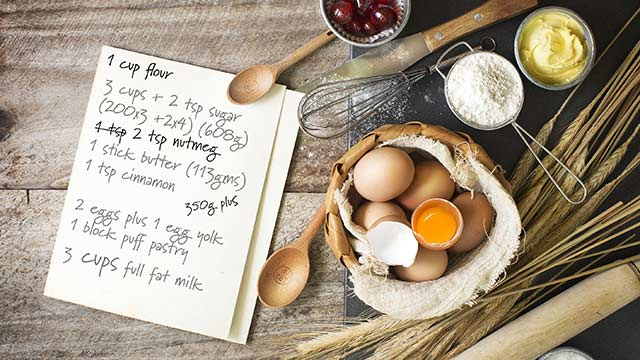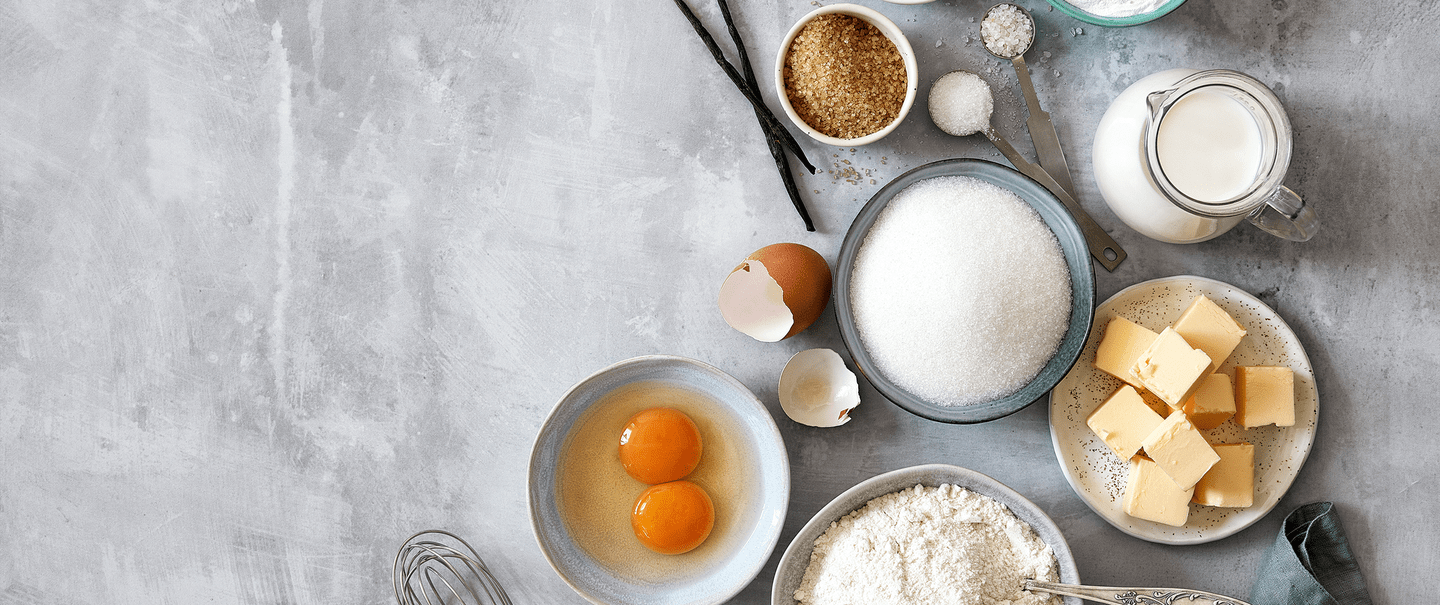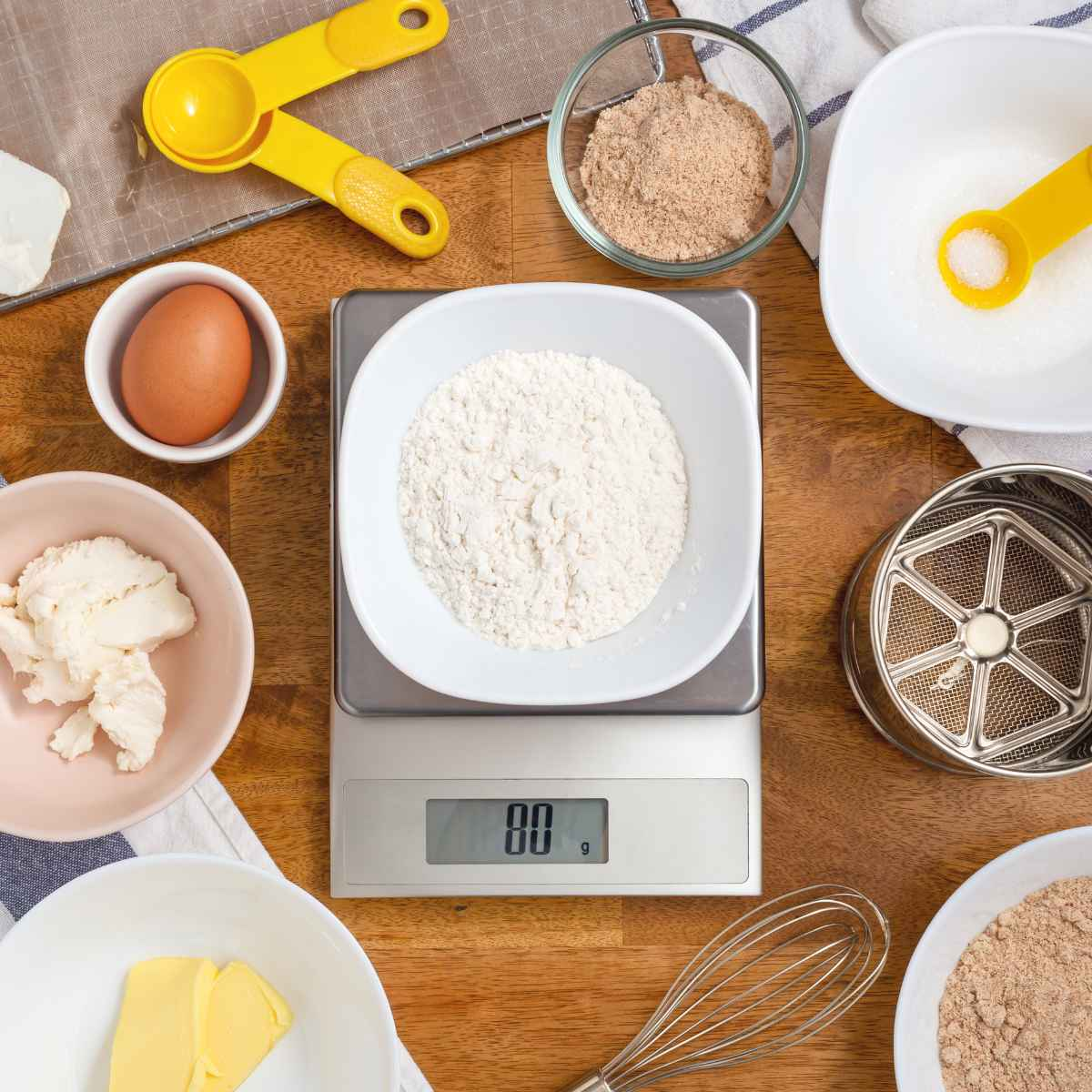## The Conversions of IngredientsConversions of Basic Ingredients from Cups to Grams & ml:

When we want to make a recipe, it´s necessary to weigh or measure the
ingredients we´ll use! For the better approach to everyday cooking, here are the
conversions of basic kitchen ingredients from cups and tablespoons, teaspoons to
grams and ml. I´ll try to categorize them and make them as readable and
understandable as possible! So, the tablets and the categories below give more
information about each ingredient! Let´s start!

Cups to ml:

• 1 cup= 250 ml
• ½ cup=125 ml
• 1/3 cup= 80 ml
• ¼ cup= 60 ml
• ¾ cup= 180 ml
• 2/3 cup= 160 ml
• 4 cups= 1000 ml / 1 L

Some examples of the basic ingredients:Liquids (water, oil, vinegar, orange juice):
• 1 cup= 240 grams
• ½= 120 grams
• ¼= 60 grams
• 2/3= 160 grams
• 3/4= 180 grams

Milk:
• 1 cup= 245 grams
• ½= 120 grams
• ¼= 70 grams
• 2/3= 165 grams
• ¾= 180 grams

Heavy Cream:
• 1 cup= 235 grams
• ½= 115 grams
• 1/3= 80 grams
• ¼= 60 grams
• 2/3= 115 grams
• 1 tbsp= 15 grams

Butter:
• 1 cup= 227 grams
• ½= 113 grams
• 1/3= 76 grams
• ¼= 57 grams
• 1 stick of butter= 114 grams
• ½ stick of butter= 56 grams

Eggs:
• 1 big egg= 70 grams
• 1 medium egg= 60 grams
• 1 small egg= 50 grams
• 1 egg yolk= 18-20 grams
• 1 egg white= 28-30 grams

Flour / Powder Sugar:
• 1 cup= 125 grams
• ½= 65 grams
• 1/3= 40 grams
• 1/8= 2 tbsps.

Cacao Powder:
• 1 cup= 125 grams
• ½= 60 grams
• ¼= 30 grams
• 2/3= 80 grams
• ¾= 95 grams

Oats / Grains:
• 1 cup= 85 grams
• ½= 43 grams
• 1/3= 28 grams
• ¼= 21 grams

Granulated Sugar:
• 1 cup= 200 grams
• ½= 100 grams
• 1/3= 67 grams
• ¼= 50 grams
• 2/3= 134 grams
• ¾= 150 grams
• 2 tbsps.= 25 grams

Brown sugar:
• 1 cup= 220 grams
• ½= 110 grams
• 1/3= 73 grams
• ¼= 55 grams

Honey / Sirups:
• 1 cup= 340 grams
• ½= 170 grams
• 1/3= 113 grams
• ¼= 85 grams
• 2/3= 227 grams
• ¾= 255 grams
• 2 tbsps.= 43 grams

Some Extra ingredients:• 1 cup of grated walnuts= 125 grams
• 1 cup almond flour= 150 grams
• 1 cup of breadcrumbs/Panko= 105 grams
• 1 cup of grated cheese= 110 grams
• 1 cup of grated coconut= 90 grams
• 1 cup of corn starch= 120 grams
• 1 cup of whipped cream= 125 grams
• 1 cup of semolina= 170 grams
• 1 cup of nuts= 150 grams
• 1 cup of lemon juice= 250 grams
• 1 cup of coconut oil=224 grams
• 1 cup of cheese cream= 225 grams
• 1 cup of sour cream= 240 grams
• 1 cup of Greek yogurt= 230 grams
• 1cup of sweetened milk=312 grams
• 1 cup of white rice= 225 grams
• 1 tbsp of baking powder= 15 grams
• 1 tbsp of baking soda= 15 grams
• 1 tbsp of dry yeast= 12 grams
• 1 tbsp of gelatin= 8 leaves of gelatin
• 1 tbsp of salt= 18 grams

Tbsps to cups:

• 4 tbsps= ¼ cup
• 5 tbsps= 1/3 cup
• 8 tbsps= ½ cup
• 10 tbsps= 2/3 cup
• 12 tbsps= ¾ cup
• 16 tbsps= 1 cupNotes: 1 cup of non-sifted flour= 140 grams. First, you should sift the flour (such
as the most of the powders) and then measure it! 1 cup of sifted flour= 1 cup
non-sifted flour minus 1 & ½ tbsps.

Dry yeast & fresh yeast= 1/3. It means, when a recipe writes for example 10 gr of
dry yeast (1 pack), you´ll add 30 gr of fresh yeast!

Enjoy!

Eleni xx